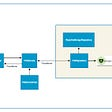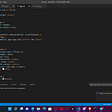# Learning to Code: Day 51 — Basic JavaScript Part 16

Hello everyone, I hope you are all keeping well. We’re almost there with JavaScript Loops, we’re heading into chapter 89, FreeCodeCamp does not mess around! Thanks to them for the lessons.

## JavaScript Do..While Loops

Another type of loop that we can work with is a do..while loop, and basically takes the framework of a while loop and turns the execution order of its condition and statement around. So, where a while loop says “while this is happening (condition), do this (statement)..” and do while loop says “do this (statement)… while this (condition) is happening”

So, the loop executes the do part, the statement, no matter what, and then loops the condition (i < 5) as long as it remains true. In this example, it will add values in the array in accordance with the do part of the loop, and give us an answer of  as it has pushed through one successful loop no matter what. If this were the other way around it would look like so:

The only difference here is the order of the code as above, but in this case of a normal while loop, here the condition is passed first, therefore i == 5 and not smaller (which in turn makes the condition i < 5 turn false), and so the array is given no values and remains empty as [].

So therefore, a do..while loop ensures that a condition will be passed through the loop at least once.

## Replacing Loops with Recursion

Recursion, in JavaScript, is the concept that a function can be expressed in terms of itself. Let’s fully understand the term:

Recursion occurs when a thing is defined in terms of itself or of its type. Recursion is used in a variety of disciplines ranging from linguistics to logic. The most common application of recursion is in mathematics and computer science, where a function being defined is applied within its own definition.

- Wikipedia.

Let’s think about the following task: Multiply the first (n) elements of an array to create the product of those elements. In a loop, that would look like this:

However, notice here that multiply(arr, n) is the same as (or ==) the following: multiply(arr, n - 1) * arr[n - 1].

From here, we can rewrite the multiply function with recursion, and avoid using a loop at all:

So here, we see in the if statement that where n <= 0, (the base case), it returns 1. In the else statement, for larger values of n, the function calls itself, but with a slight moderation of “n - 1”. That call is evaluated in the same way calling a multiply until n <= 0. At this point in the execution, all functions can return and the original multiply returns the answer.

Note: Recursive functions must include a base case otherwise they will never end.

## Profile Lookup Challenge

Okay let’s look at an array of objects and how we can apply a for loop to a function to figure out two if statements (seen below). Here’s the setup:

We have here one object called contacts, one top-level array with 4 nested objects inside (later on, each is referred to as i), each containing various key and value metadata and another nested array also.

We then ask ourselves a set of questions set out by FreeCodeCamp:

The function should check if `name` is an actual contact's `firstName` and the given property (`prop`) is a property of that contact.

If both are true, then return the “value” of that property.

If `name` does not correspond to any contacts then return `"No such contact"`.

If `prop` does not correspond to any valid properties of a contact found to match `name` then return `"No such property"`.

Now how do these questions look in the code? Let’s take a look-see:

We start with a for loop on line 31 followed by the two if statements on line 32 and 33.

Note: Instead of using the .hasOwnProperty() method to check for prop, we use the `in` operator on line 33. This is effectively saying “if prop is in contacts array number x..”

This is rather logical to work through, however a couple of new things here. We’re looking at using a for loop within a function instead of writing out an if/else statement. The second if statement is nested within the first. It’s also worthy noting here that [i] accounts for the object index within the top-level array.

Aaaand let’s call it there before it gets too out of hand! Thanks guys for checking in and I’ll see you next time!

--

--

--

## More from The Startup

Get smarter at building your thing. Follow to join The Startup’s +8 million monthly readers & +756K followers.

## Web Share API test## Processing streaming data with Spring WebFlux## 3. First steps in React## Build a Mini Buymeacoffee dApp Using Solidity, Ethereum Smart Contract, React and TailwindCSS## How to set up functional testing for a web app from scratch in 5 minutes## Schedule Background Tasks in React Native App## Killer JavaScript One-Liners ☝️## Hugh Burgess

Step by step, learning to code with FreeCodeCamp

## The Need for TypeScript-first Beginner Learning Content## Coding Bootcamp Week 9/13: New Year, New Tech## Pair Programming